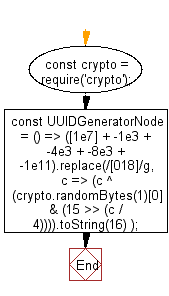# JavaScript: Generate a UUID in Node.JS.

## JavaScript fundamental (ES6 Syntax): Exercise-254 with Solution

Write a JavaScript program to generate a UUID in Node.JS. Use crypto API to generate a UUID, compliant with RFC4122 version 4.

• Use crypto.randomBytes() to generate a UUID, compliant with RFC4122 version 4.
• Use Number.prototype.toString(16) to convert it to a proper UUID.

Sample Solution:

JavaScript Code:

``````//#Source https://bit.ly/2neWfJ2
const crypto = require('crypto');
const UUIDGeneratorNode = () =>
([1e7] + -1e3 + -4e3 + -8e3 + -1e11).replace(//g, c =>
(c ^ (crypto.randomBytes(1) & (15 >> (c / 4)))).toString(16)
);
console.log(UUIDGeneratorNode());
```
```

Flowchart:Improve this sample solution and post your code through Disqus

What is the difficulty level of this exercise?

Test your Programming skills with w3resource's quiz.

﻿